• 文章目录传递函数由来MATLAB simulink子系统封装simulink子系统mask绘制完整流程图--运行显示绘制子系统中传递函数的bode图、nyquist图、step图等修改参数后的两个图相比较： 传递函数由来 MATLAB simulink子系统...
文章目录传递函数由来MATLAB simulink子系统封装simulink子系统mask绘制完整流程图--运行显示绘制子系统中传递函数的bode图、nyquist图、step图等修改参数后的两个图相比较：
传递函数由来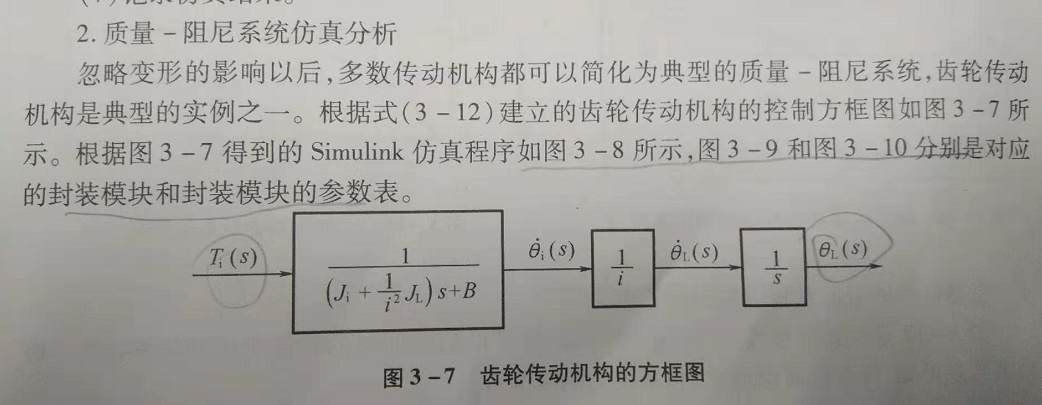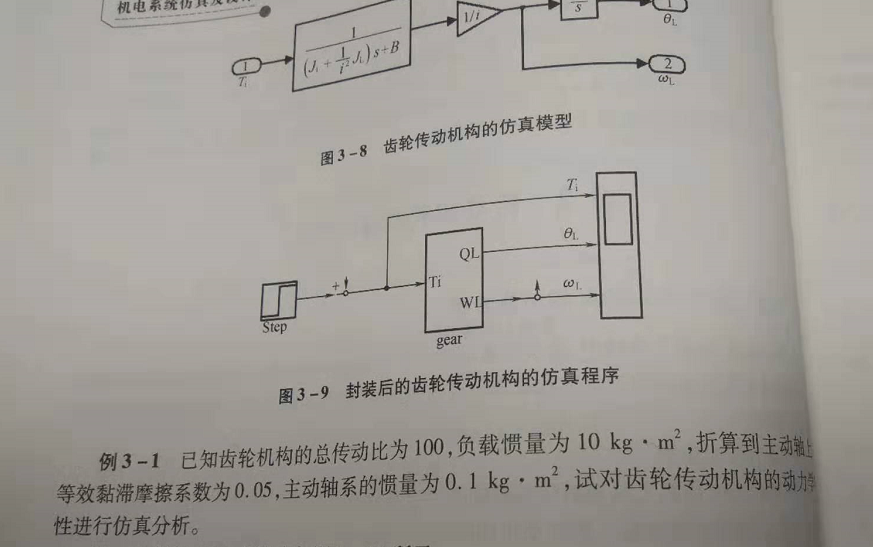MATLAB simulink子系统封装
在simulink中新建流程图：
如下图所示：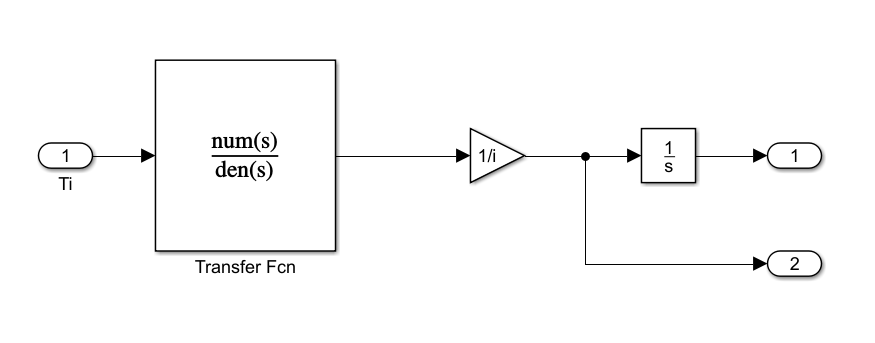参数设置：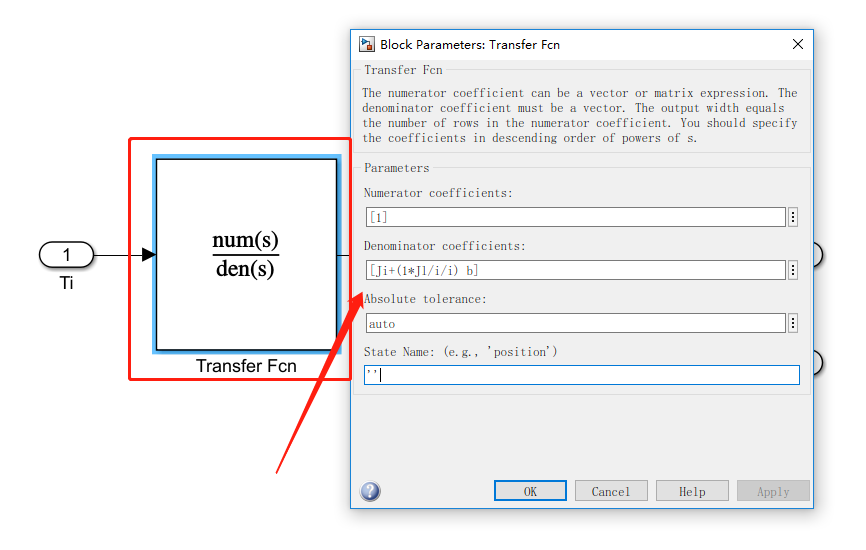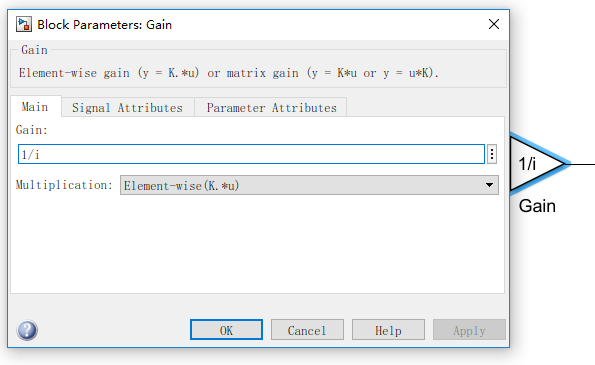选择后—全选CTRL+A，然后CTRL+G封装成子系统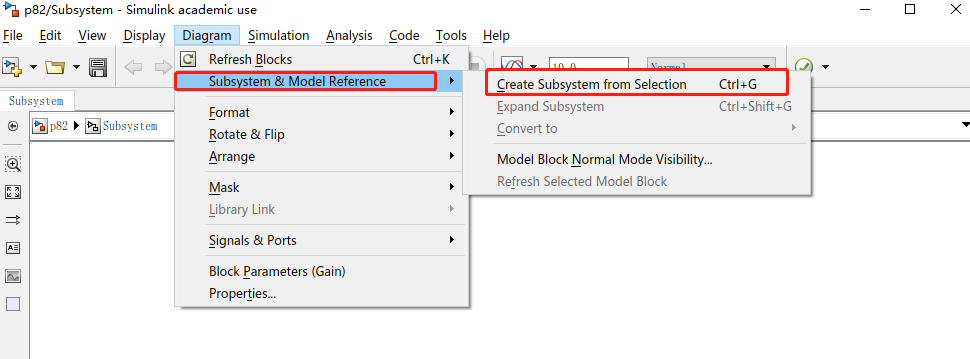simulink子系统mask
右键mask–editmask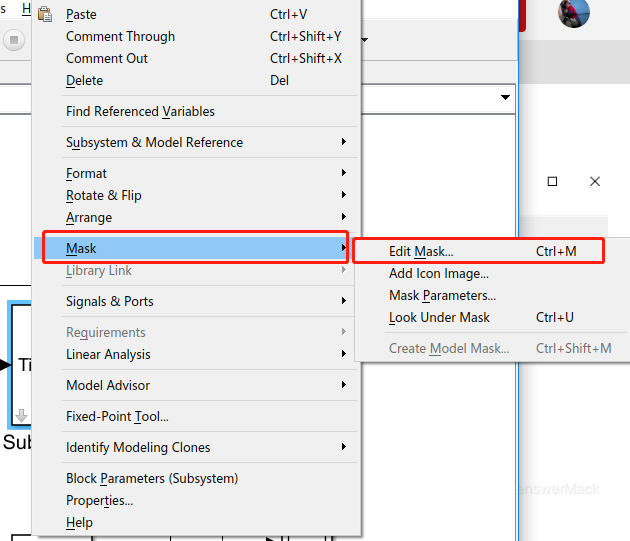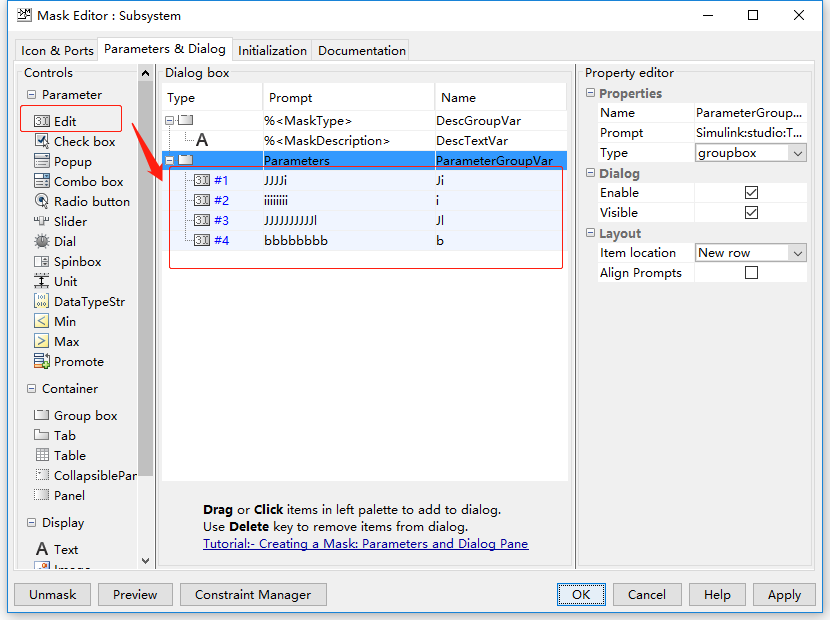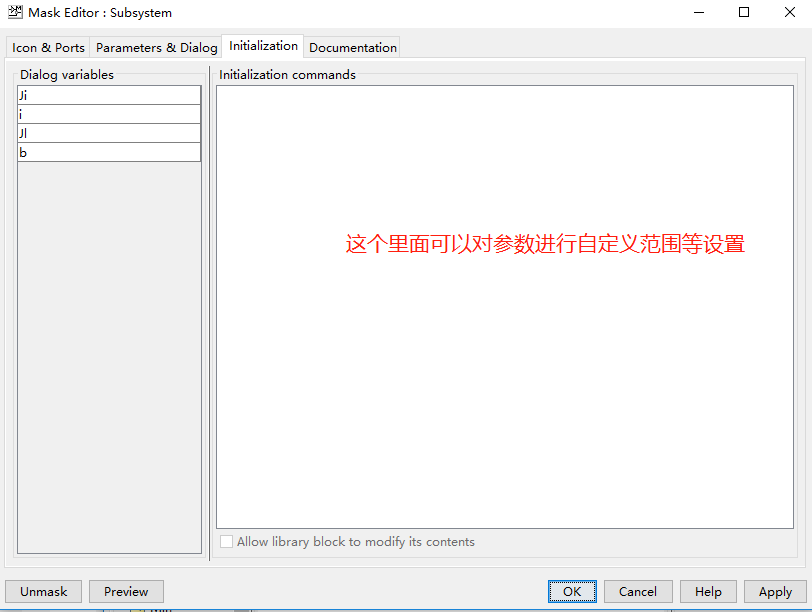绘制完整流程图–运行显示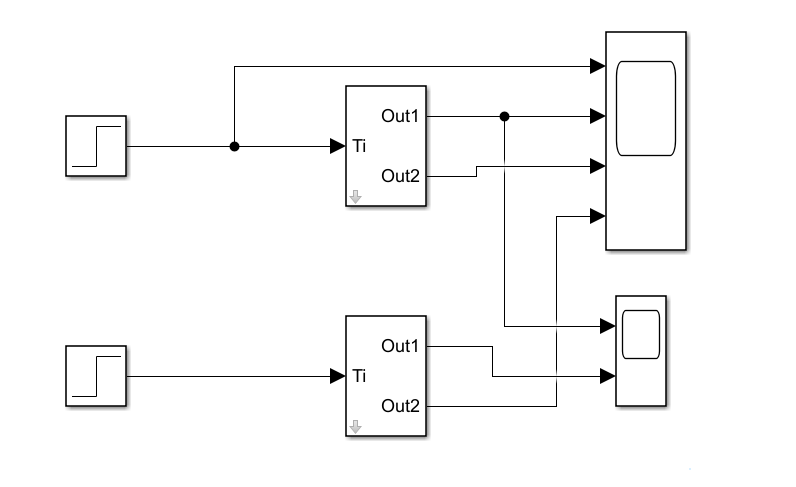设置参数：
双击子系统块设置参数值：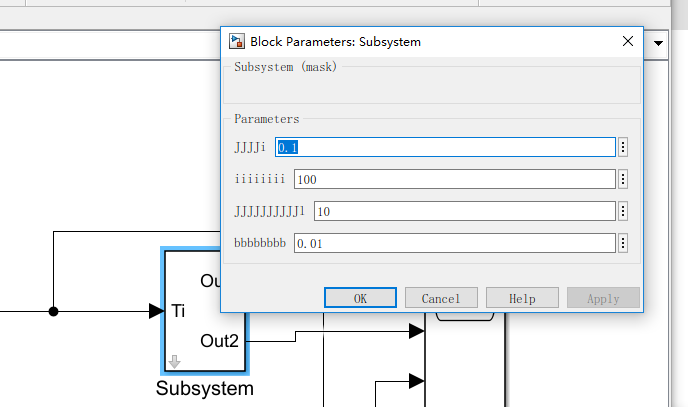运行显示。在view视图的layout中选择显示图框
选择legend中✔，可以在图框中看到曲线是哪个输出。

两条曲线相比较可以采用在同一个scope中显示的方法。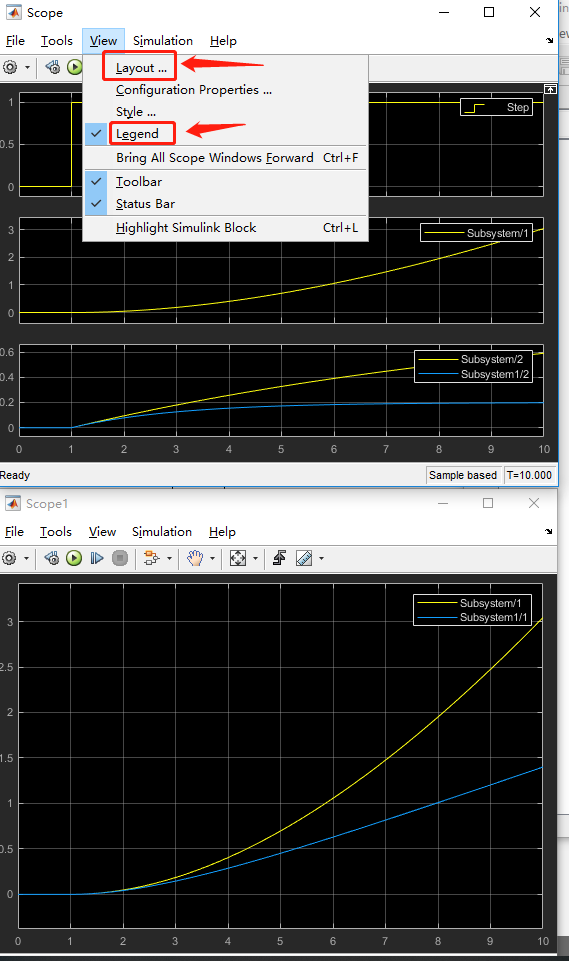绘制子系统中传递函数的bode图、nyquist图、step图等
在子系统中CTRL+U进入子系统项：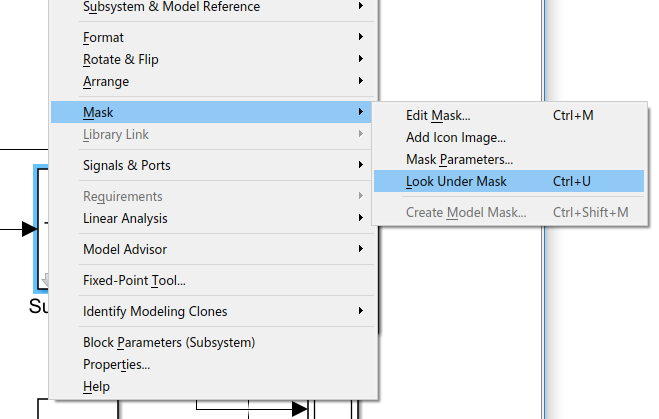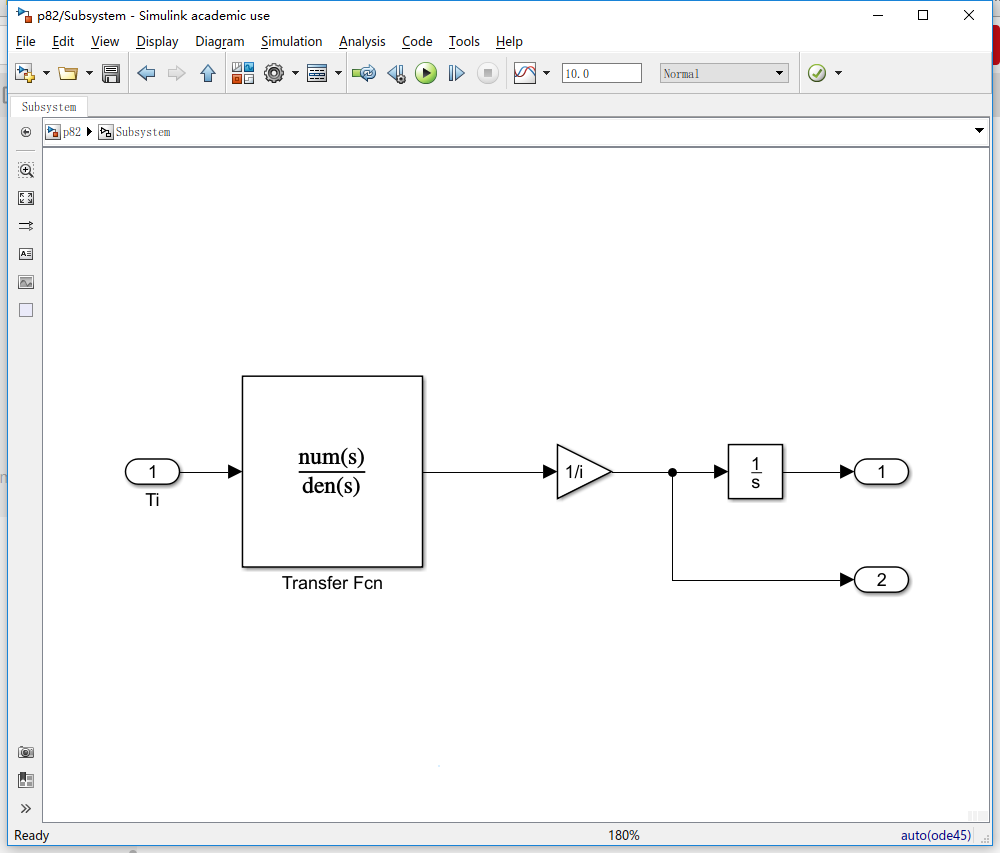选中传递函数 右键选择linear analysis–linearize block：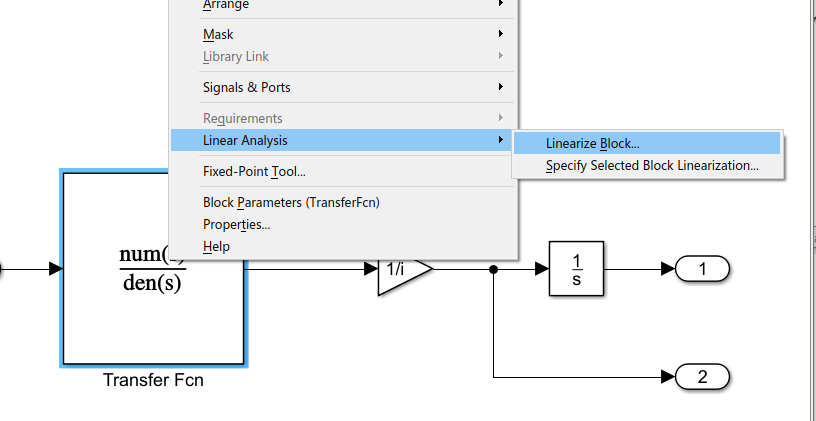绘制BODE图：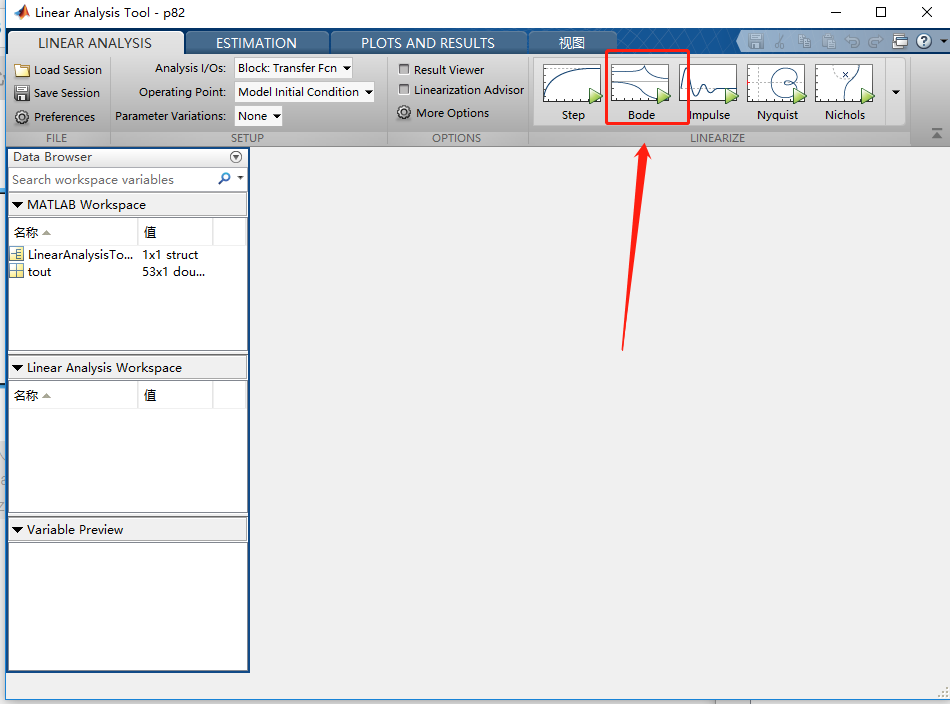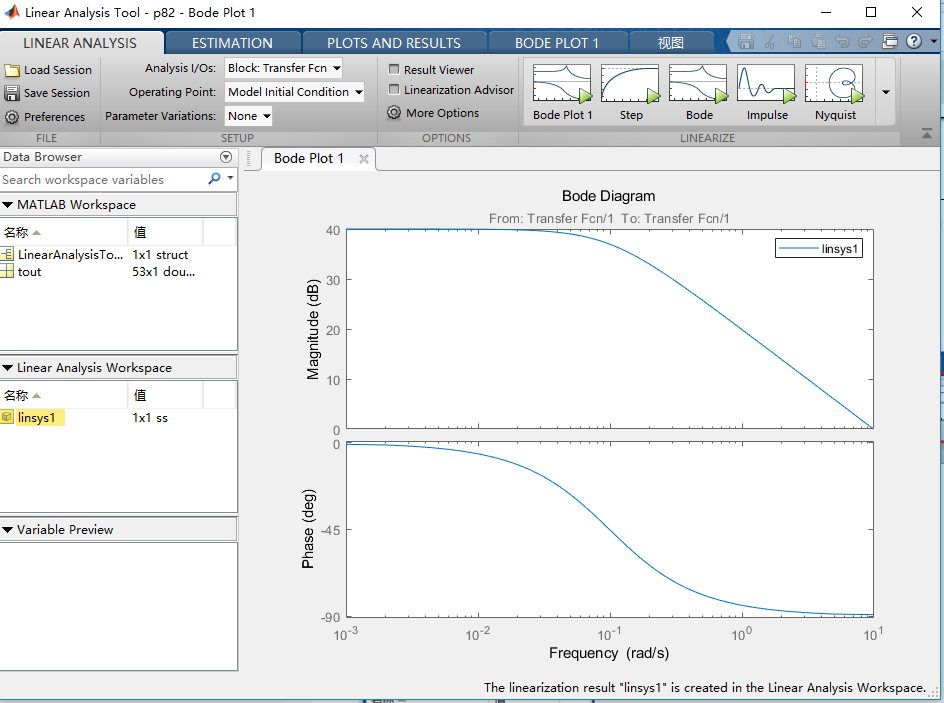修改参数再次绘制bode图：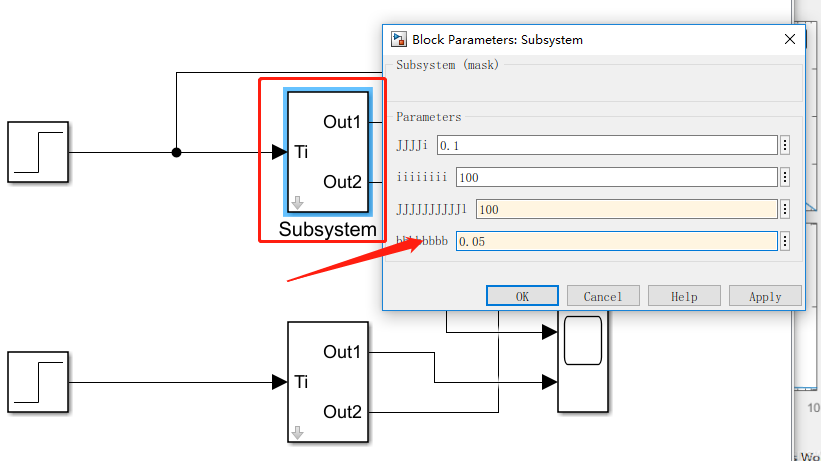再选BODE图：此时生成幅值图和相位图：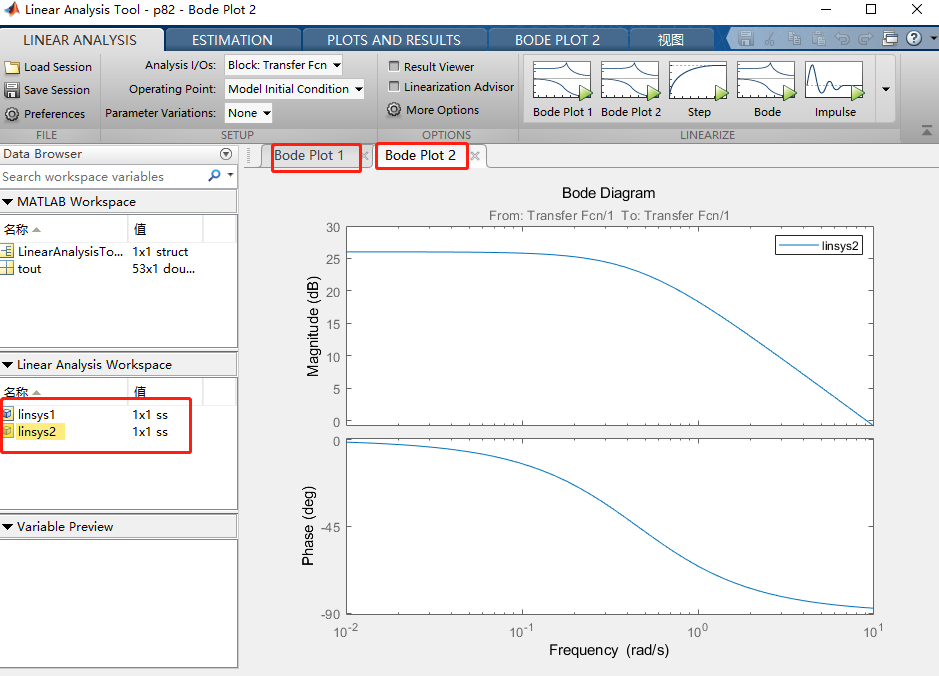修改参数后的两个图相比较：
手动将linsys2的值拖入到bode plot1中：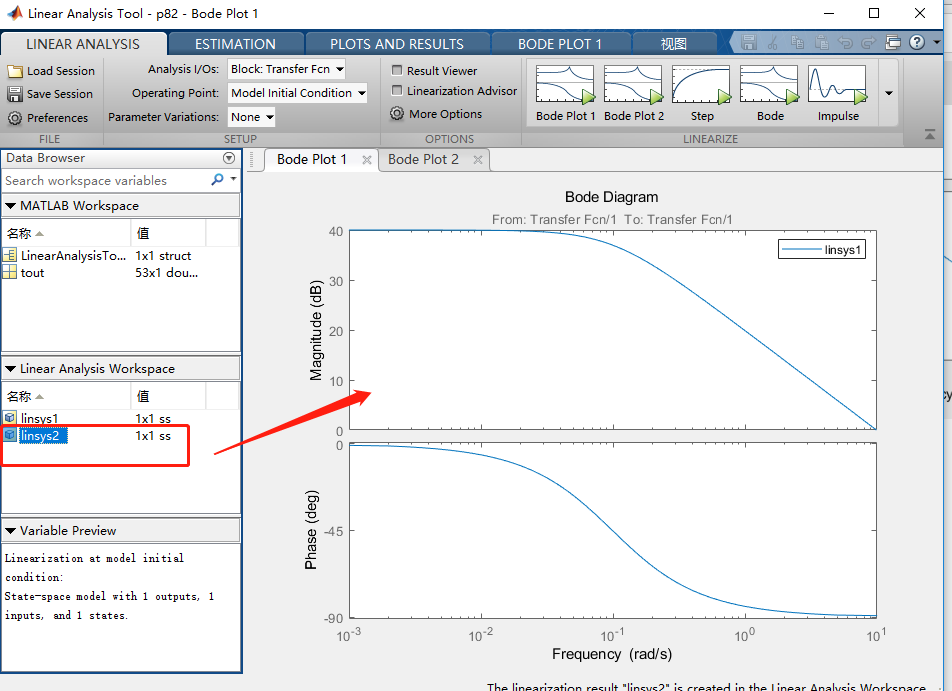得到：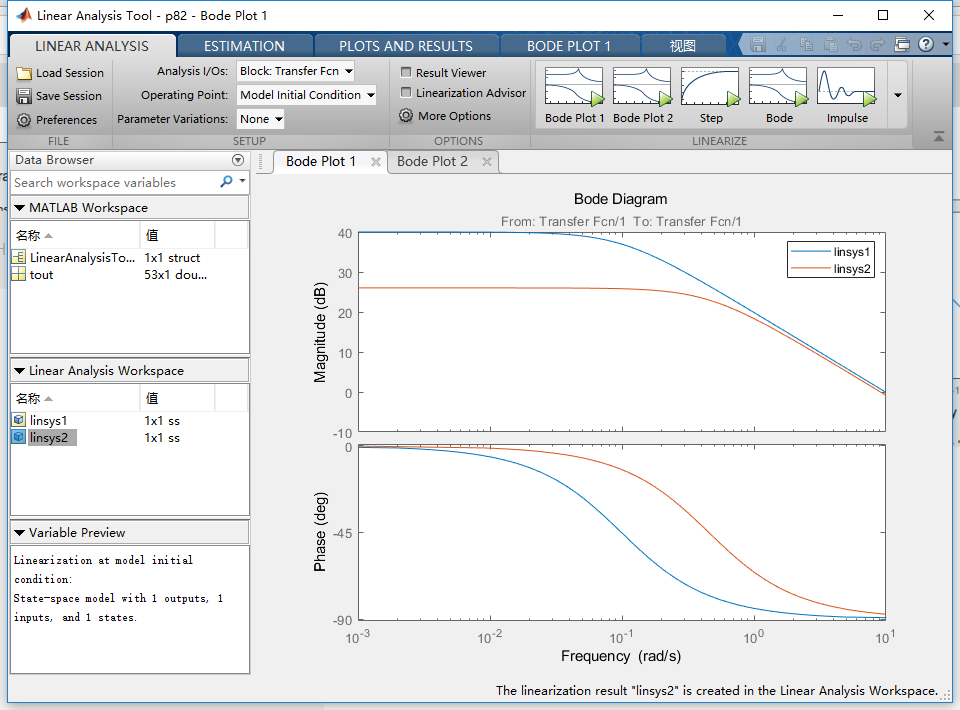同理绘制其他图形！！！！！！！！
小细节自行查阅help文档。
参考文档：
https://ww2.mathworks.cn/help/simulink/block-masks.html   官方


展开全文simulink
• 函数需要另一个 FEX 函数：contourcs ( http://www.mathworks.com/matlabcentral/fileexchange/28447 ) 例子： mask = imread('circuit.tif')>100; P = mask2poly(mask); 数字; 图像（掩码）； 轴在； 开箱； ...
• 它是 Matlab poly2mask 函数的一种补充。 不同之处在于返回所有轮廓点 - 没有丢失线性相关点的点。 为了获得 poly2mask 的 100% 互补，应省略直线内的所有点。 就我而言，我实际上需要所有这些点，作为投资回报率的...
• Matlab中很多函数我们用起来很方便，比如：imhmax，imhmin，imreconstruct，imregionalmin。这个代码就是基于opencv实现Matlab函数的 cv::Mat imhmax(cv::Mat im, double thresh); cv::Mat imhmin(cv::Mat im, ...
• <p>Hello everyone please i need help with this issue.I have a matlab function that returns a value 'pourcentage' i want to retrieve this value in php file . here is my function <pre><code> function ...php
• matlab源码求一元函数 .../matlab/function/mask：自适应掩蔽因子相关函数 /matlab/function/tool：一些工具函数 /matlab/function/transform：变换函数，主要是 DFRNT 变换簇和 DFRFT 变换簇 /matlab/functi
• 本文基于信息光学初等函数及基础几何图形学，用Matlab定义并编写得到几何图形掩膜的函数。 Project Code 掩膜主函数定义如下： % 作者：ZQJ % 日期：2021.1.29 星期五 %***********************几何图形掩膜函数...
本文基于信息光学初等函数及基础几何图形学，用Matlab定义并编写得到几何图形掩膜的函数。

Fouction Code

掩膜主函数定义如下：

% 作者：ZQJ
% 日期：2021.1.29 星期五

%***********************几何图形掩膜函数**************************
function mask_ = Z_Masks
mask_.single_silt    = @mask1;
mask_.rect_hole      = @mask2;
mask_.circle_hole    = @mask3;
mask_.cir_srceen     = @mask4;
mask_.triangle_hole  = @mask5;
end

单狭缝掩膜

基于一维矩形函数，得到单狭缝掩膜；
函数定义和代码如下：

function my_mask = mask1(m_unit_width,N,silt_width)
% 函数功能：利用一维矩形函数产生单狭缝掩膜
% 参数
% m_unit_width：掩膜的单位像素宽度
% N：一维方向上的像素点个数（例1024即1024x1024）
% silt_width：单狭缝的宽度

mask_x = -m_unit_width*N/2 : m_unit_width : m_unit_width*N/2;
mask_y = rectpuls(mask_x,silt_width);
% plot(mask_x,mask_y);
[mask_column,~] = meshgrid(mask_y);
my_mask = mask_column;
end

矩形孔掩膜

基于二维矩形函数，得到矩形孔掩膜；
函数定义和代码如下：

function my_mask = mask2(m_unit_width,N,rect_width,rect_height)
% 函数功能：利用二维矩形函数产生矩形孔掩膜
% 参数
% m_unit_width：掩膜的单位像素宽度
% N：一维方向上的像素点个数（例1024即1024x1024）
% rect_width：矩形孔的宽度
% rect_height：矩形孔的高度

mask_x = -m_unit_width*N/2 : m_unit_width : m_unit_width*N/2;
[mask_x_axis,mask_y_axis] = meshgrid(mask_x);
mask_z_axis = rectpuls(mask_x_axis,rect_width).*rectpuls(mask_y_axis,rect_height);
my_mask = mask_z_axis;
end

圆孔掩膜

基于基础几何图形理论，得到圆孔掩膜；
函数定义和代码如下：

function my_mask = mask3(m_unit_width,N,cir_R)
% 函数功能：产生圆孔掩膜
% 参数
% m_unit_width：掩膜的单位像素宽度
% N：一维方向上的像素点个数（例1024即1024x1024）
% cir_R：圆孔掩膜的半径

mask_x = linspace(-m_unit_width*N/2,m_unit_width*N/2,N);
[mask_x_axis,mask_y_axis] = meshgrid(mask_x);
my_mask = zeros(N);
my_mask(abs(mask_x_axis + 1i*mask_y_axis) < cir_R) = 1;
end

圆屏掩膜

基于基础几何图形理论，得到圆屏掩膜；
函数定义和代码如下：

function my_mask = mask4(m_unit_width,N,cir_R)
% 函数功能：产生圆屏掩膜
% 参数
% m_unit_width：掩膜的单位像素宽度
% N：一维方向上的像素点个数（例1024即1024x1024）
% cir_R：掩膜圆屏的半径

mask_x = linspace(-m_unit_width*N/2,m_unit_width*N/2,N);
[mask_x_axis,mask_y_axis] = meshgrid(mask_x);
my_mask = ones(N);
my_mask(abs(mask_x_axis + 1i*mask_y_axis) < cir_R) = 0;
end

三角孔掩膜

基于基础几何图形理论，得到三角孔掩膜；
函数定义和代码如下：

function my_mask = mask5(m_unit_width,N,triangle_a)
% 函数功能：产生三角孔掩膜(近似等边三角形)
% 参数
% m_unit_width：掩膜的单位像素宽度
% N：一维方向上的像素点个数（例1024即1024x1024）
% triangle_a：三角形的边长为m_unit_width的倍数

my_mask = zeros(N);
r = double(uint8(triangle_a*m_unit_width/3));
for col = (m_unit_width*(N/2-triangle_a)+1):m_unit_width:(m_unit_width*(N/2+triangle_a)+1)  %定义扫描区域
for row = (m_unit_width*(N/2-triangle_a)+1):m_unit_width:(m_unit_width*N/2+1)
x = col - (m_unit_width*N/2+1);
y = (m_unit_width*N/2+1) - row;
if(y <= (sqrt(3)* x + m_unit_width*triangle_a) && y <= (-sqrt(3)* x + m_unit_width*triangle_a)) %60°倾角
my_mask((row+r),col) = 1;
end
end
end
end

仿真结果图：

单狭缝掩膜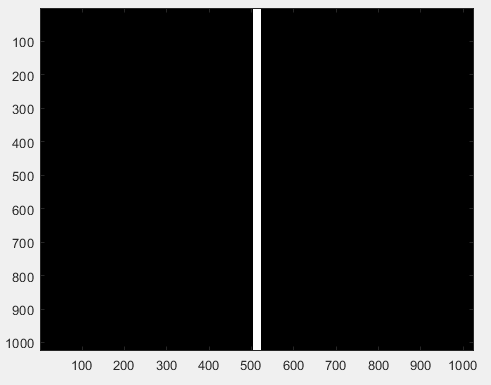矩形孔掩膜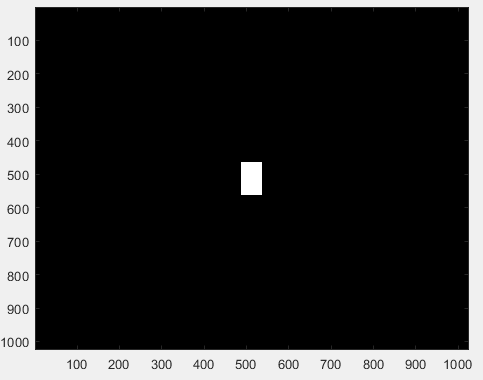圆孔掩膜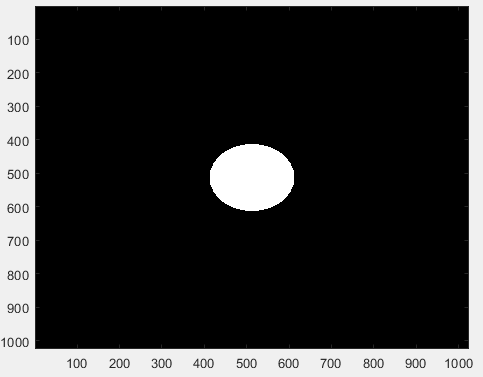圆屏掩膜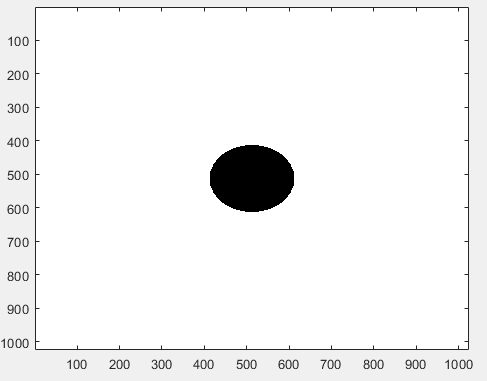三角孔掩膜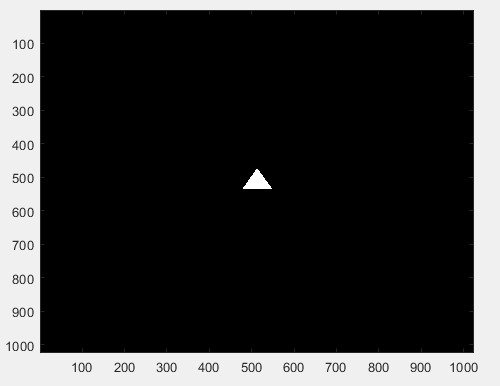专栏内容供作者本人或大家学习使用，多多指教 ~


展开全文• Matlab函数的集合，用于对fMRI数据（例如静止或稳态数据）进行降噪。 fmri_cleaning通过单个线性回归模型执行数据降噪-包括频率滤波，去除不感兴趣的信号（例如，运动或生理估计）以及检查运动污染的体积。 这是主要...
• matlab的运算主要有算术运算、逻辑运算和比较运算。再次不作过多的说明，主要是在matlab使用过程中的一些注意事项。如上所示，x(mask)所取出的是逻辑数组mask中为真的部分。因此x（mask）=1表示的是将其中mask为真的...
matlab的运算主要有算术运算、逻辑运算和比较运算。再次不作过多的说明，主要是在matlab使用过程中的一些注意事项。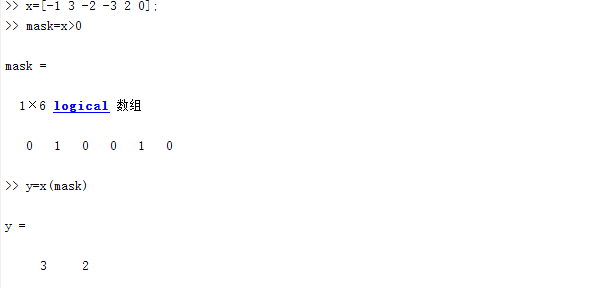如上所示，x(mask)所取出的是逻辑数组mask中为真的部分。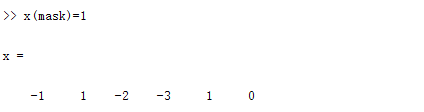因此x（mask）=1表示的是将其中mask为真的部分的值赋值为1；此处与find()函数的作用类似：find（）查找非 零元素的索引。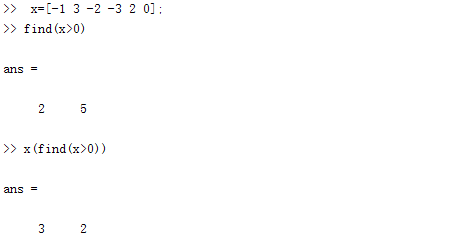结果与上面使用逻辑数组的表现是一样的。逻辑函数        ischar():如果元素中包含字符，则返回1；        isinf():如果()中有infinity（inf），则返回1；        isnumerical():如果()中含有数字，则返回1；        isempty():如果（）中含有空数组（x=[]）,则返回1；取整函数        round（x）：对于x中的所有元素按照四舍五入（离那个整数近就往那边取整）的方式取整。        fix（x）:对于x中的所有元素朝零的方向取整。        ceil（x）：对于x中的元素向上取整，即取得大于x的最小整数。        floor（x）：对于x中的所有元素向下取整，即取得小于x的最大整数。数据分析函数        max（x）:求数组x中的最大元素。        min（x）：求数组x中的最小元素。        mean（x）：求得数组元素的均值。        sum（x）：求数组元素的和。        prod（x）：求数组元素的乘积。        sort（x）：对x中的元素进行升序排列。如果为矩阵，则将矩阵中的每列元素按列向量排序。        sort（x）：对x中的元素进行行排列。与sort中默认的相反。        find（x）：查找并返回非零元素的索引和值。        size（x）：返回一个行向量，包含x的行列维度。        length（x）：返回x中的最大维度。        complex(x,y)：创建复数数组。其中x，y可以是数组，但是其维度必须相同。随机均布函数        x=rand;返回一个在(0,1)之间均匀分布的随机数。        x=rand(n);返回一个n*n的随机数的矩阵，矩阵的元素在(0,1)之间的随机数。        x=rand(size1,size2);返回一个矩阵，其维度为size1、size2.元素值为(0,1)之间。        x=randi(max);返回一个介于1和max之间的随机整数。        x=randi(max,n)；与rand(n)一样，只不过max规定了其取值范围为(1,max)之间的随机整数。        x=randi(max,size1,size2)；与x=rand(size1,size2);和 x=randi(max);合并的结果相同。        x=randn;返回一个从标准正态分布中得到的随机标量。其余的与rand和randi类似，在此不再说明。其他函数         clock：当前日期和时间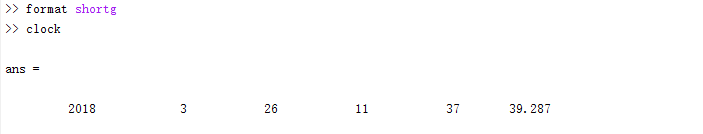date：当前日期；以字符串的形式显示。（格式：day-month-year）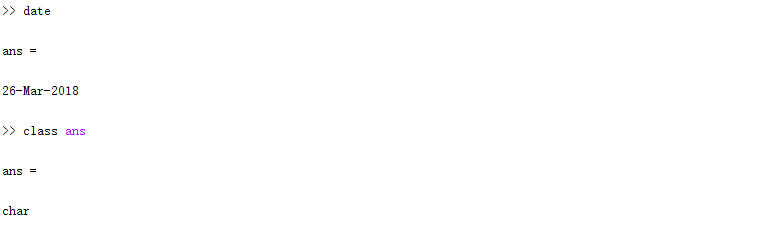tic和toc：tic表示启用秒表计时器，该命令会记录tic内部的命令执行时间。使用top函数显示已用时间。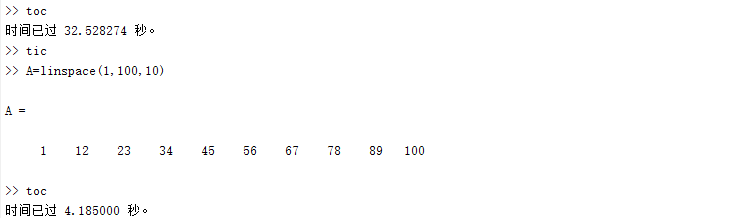pause：暂停执行MATLAB语句;并等待用户按下任意键。        pause（n）：暂停执行n秒
展开全文• 自定义函数实现对灰度图像的均值滤波，要求对用户输入的掩模（mask）进行检查： 1. 掩模必须是方形（行列数量相等） 2. 掩模边长必须是奇数 3. 掩模必须关于中心对称 另外，对原图进行边缘补0。 要求：输入图像...
题目

自定义函数实现对灰度图像的均值滤波，要求对用户输入的掩模（mask）进行检查：
1. 掩模必须是方形（行列数量相等）
2. 掩模边长必须是奇数
3. 掩模必须关于中心对称
另外，对原图进行边缘补0。
要求：输入图像和输出图像的大小和数据类型必须相同。

函数原型：
function [filteredIm] = AverageFiltering (im, mask)
im 为输入图像
mask 为输入掩模
filteredIm 为输出图像

代码
1. AverageFiltering 函数代码

function [filteredIm] = AverageFiltering (im, mask)

% To check whether mask is qualified
[rowM, colM] = size(mask);
if rowM ~= colM
disp 'The mask has to be square!';
return
end
if mod(rowM,2) == 0
disp 'The mask’s dimension has to be an odd number!';
return
end
A = mask;
if ~isequal(mask, rot90(A,2))
disp 'The mask has to be symmetric around the center!';
return
end

% To filter the input image
[row, col] = size(im);
doubleIm = double(im);

% Because I will use (rowM-1)/2 frequently
% After padding 0 new matrix's size will be (M+w-1)*(N+w-1) where w is the length of mask
padding = (rowM-1)/2;

% Assign the padding as 0
temp1 = zeros(row+rowM-1,col+colM-1); % All zero
temp1(padding+1:padding+row, padding+1:padding+col) = doubleIm;
temp2 = temp1;

% only apply to those who are not 0
for i = padding+1 : padding+row
for j = padding+1 : padding+col
cover = temp1(i-padding:i+padding,j-padding:j+padding).* mask; % get rowM*colM matrix to .* mask
sumValue = sum(sum(cover)); % get the sum of those rowM*colM numbers
temp2(i,j) = sumValue /(rowM * colM);
end
end

% return the processed image
filteredIm = uint8(temp2(padding+1 : padding+row, padding+1 : padding+col));  

2.主函数代码

% load image
circurt = imread('Circuit.jpg');

% call AverageFiltering to process circuit with different mask
circuitAF1 = AverageFiltering(circurt,[1 2 1;2 4 2;1 2 1]);
circuitAF2 = AverageFiltering(circurt,ones(5));
% show images
figure(1);
subplot(1,3,1); imshow(circurt);title('orginal');
subplot(1,3,2); imshow(circuitAF1);title('weighted mask');
subplot(1,3,3); imshow(circuitAF2);title('standard mask');

运行结果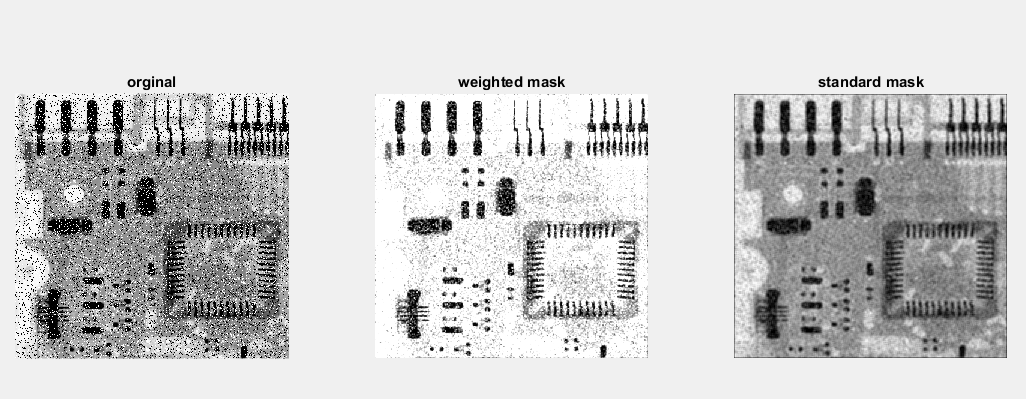展开全文均值滤波
• 描述：以mask为约束，连续膨胀marker，实现形态学重建，其中mask >= marker 参数： - marker 标记图像，单通道/三通道图像 - mask 模板图像，与marker同型 - conn 联通性重建结构元，参照m
• [imo,mask] = rbfwarp2d(im, ps, pd, 方法) 输入： im：图像二维矩阵ps：二维源地标[n*2] pd: 2d destin 地标 [n*2] 方法： 'gau',r - 对于高斯函数 ko = exp(-|pi-pj|/r.^2); 'thin' - 对于薄板函数 ko = (|pi-pj|...
• matlab缩放函数图像代码单发MultiBox检测器 注意：此存储库不再维护。 对于主动维护的易于使用的物体检测器，我建议考虑使用PyTorch Mask R-CNN。 该目录包含用于训练和评估本文所述的SSD对象检测器的代码： SSD: ...
• 在数字图像处理过程中常会用到形态学处理的函数，比如膨胀和腐蚀等操作，在次之前往往需要创建一个掩膜（mask），本文仿照matlab的strel函数实现了OpenCV下的近似版本。opencv 图像处理
• mask = torch.tensor(mask) #pytroch中要输入tensor，mask原来为numpy，转化成tensor print(mask.shape,'初始的mask维度') mask = mask.unsqueeze(-1) #在最后增添一维 print(mask.shape,'增加一个维度以后'python pytorch
• 1、Poly2mask-----------------------------------------------------将感兴趣的区域转换成掩膜 bw=poly2mask(x,y,m,n)-------------讲一个ROI多边形转黄成二值图像的掩膜区域，向量x y分别表示ROI多边形个顶点x y...
• 此代码将像素位置转换为其质心坐标。 它适用于 DICOM 多切片图像，其中每个体素都是一个立方... 该函数取 INPUT：VoxDim=体素尺寸，单位为微米； name_mask='MaskName.dcm'。 它提供每个像素质心的 xyz 坐标作为 OUTPUT
• - 任何 MATLAB 内置函数，作为字符串 ('jet') 或数组 (jet(64)) 传递- 任何 Nx3 阵列- 单一颜色，在这种情况下，颜色图会扩展为从白色运行到该颜色 我将 imagesc.m 和 @double 放在我的工作目录中。 我不确定这是否...
• %output(mask==1) = y(mask==1); [PSNR_Cur,SSIM_Cur] = Cal_PSNRSSIM(im2uint8(label),output,10,10); PSNRs(i) = PSNR_Cur; SSIMs(i) = SSIM_Cur; imshow(cat(2,y,output)); drawnow; pause...testing 深度学习
• 如果你有两个多边形并且想要两个蒙版，你可以在每个多边形上分别调用 poly2mask 。 现在，假设这两个2D多边形在Z = 1和Z = 10处徘徊，并且您要沿着切片Z = 1：10制作蒙版* volume *。 为此，您需要 blendedPolyMask...
• 使用连通分量标记方法（使用 MATLAB 内置 bwlabel 函数）标记多个区域。 生成的信息： - 二进制掩码 (obj.roi) - 标签掩码（obj.labels） - 独立区域的数量（obj.number） 您可以侦听对象的“MaskDefined”事件以...
• 函数查找点 a 和 b 之间的线与二进制掩码中对象的边界之间的交点。 点a必须在对象内部。 b 点可以在图像中的任何位置。 如有必要，该函数始终通过将线延伸到点 b 之外来返回一个交点。 点 a、b 和 int 以 [x,y] ...
• ## matlab sisotool工具DEMO

千次阅读 2019-12-17 12:27:28
MATLAB simulink子系统封装mask–传递函数plot–scope显示 链接：https://blog.csdn.net/answerMack/article/details/96883836 新建一个控制框图，如下图： 利用Ctrl+G封装成子系统， 选中subsystem，按CTRL+M，...
• 我在mathworks上面复制粘贴了一个视频目标跟踪的代码，老是显示未定义函数或变量 'multiObjectTracker'。这个应该怎么解决呀 ` function MultipleObjectTrackingExample() % Create objects used for ...c语言
• matlab显示黑白图像代码...Fig1(mask).png 示例。 接下来，您必须选择液滴/基材界面的基线。 这是在至少 2 张图像上完成的，并且旋转角度是平均的。 参见 Fig2(baseline).png 示例。 裁剪和整平后，您会看到一些示例图
• ## 求连通域面积matlab

千次阅读 2019-08-30 12:28:29
matlab有自带函数bwreopen()求连通域，并移除小面积连通域，返回一个mask模板不同连通域用不同的label标志，背景用0标志。 function [ mask ] = bwareopenLZ( Lig,minarea) %UNTITLED3 此处显示有关此函数的摘要 %...
• matlab掩摸代码 ...为了易于使用，我们包含一个matlab函数functions/create_mask_fiducial.m ，该functions/create_mask_fiducial.m可计算给定关键点的蒙版。 关键点定义显示在functions/facial_la
• 笔记： - 等效的 Matlab 代码：Y = X(Mask) - 与 Matlab 的逻辑索引的区别： * 线性索引快 2 到 3 倍。 * 在每种情况下都会回复一个列向量。 * 掩码不能长于数组，而 Matlab 允许额外的尾随值，当它们为 FALSE 时。...
• matlab美白代码用于图像检索的选择性深度卷积特征 这是。中接受的Matlab实现。 该代码实现 产生全局图像表示的框架 预处理：PCA + l2归一化 屏蔽方案：MAX-mask / SUM-mask 嵌入：三角形嵌入（Temb）和快速函数...
• matlab提示输入参数的数目不足时解决办法： 方法一： 如： function [mu,mask]=kmeans(ima,k) 在命令行输入所需的参数 ： ima=imread('1.bmp');  k=2;  然后在调用这个函数  kmeans(ima,k) 方法二：  在...# mask函数matlabmatlab 订阅